在采样问题上我们可能会面对这些问题：

• 计算机只能实现对均匀分布的采样，但我们仍然可以在此基础上对更为复杂的分布进行采样，那具体该如何操作呢？
• 随机分布的某些数字特征可能需要通过积分的形式来求解，但是某些积分可能没有（或者很难求得）解析解，彼时我们该如何处理呢？
• 在贝叶斯推断中，后验概率的分布是正⽐于先验和似然函数之积的，但是先验和似然函数的乘积形式可能相对复杂，我们又该如何对这种形式复杂的分布进行采样呢？

针对这些问题衍生出一系列求解的方法。

# Inverse CDF 方法

这种方法称为逆变换采样（Inverse transform sampling）法，我们一起来回顾一下PDF和CDF。

对于随机变量 $X$，如下定义的函数 $F$

上式称为$X$的累积分布函数（CDF，Cumulative Distribution Function）。

对于连续型随机变量$X$的累积分布函数$F(x)$，如果存在一个定义在实数轴上的非负函数$f(x)$，使得对于任意实数$x$，有下式成立：

则称$f(x)$$X$的概率密度函数（PDF，Probability Density Function）。显然，当概率密度函数存在的时候，累积分布函数是概率密度函数的积分。

所以，通常我们可以通过对PDF（如下图中的左图所示为正态分布的PDF）进行积分来得到概率分布的CDF（如下图中的右图所示为正态分布的CDF）。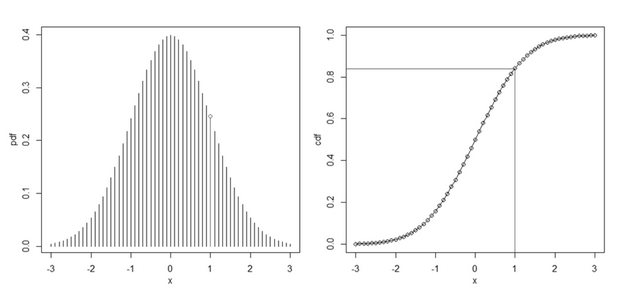我们可以求得CDF的反函数$F^{-1}(u)$，如果要得到 m 个观察值，则重复下面的步骤 m 次：

• 从 Uniform(0,1) 中随机生成一个值（前面已经说过，计算机可以实现从均匀分布中采样），用$u$表示。
• 计算$F^{-1}(u)$的值$x$，则$x$就是从$f(x)$中得出的一个采样点。

在上图中，如果从 Uniform(0,1) 中随机生成的值$u=0.8413$，则可以算得$F^{-1}(u)=1$，则此次从正态分布中生成的随机数就是 1。

为了进一步验证Inverse CDF 方法真的有效，我们从定量上算一下。

通过计算求解（python程序近期上传），我们可以拟合出真实曲线和采样绘制出的曲线：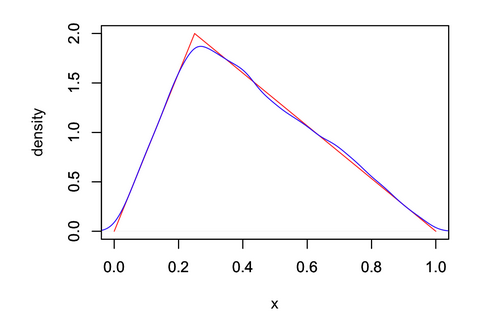从图中可以看出，采样点与原始分布非常吻合。

# 拒绝采样（Reject Sampling）

从上述描述中可以知道Inverse CDF 方法确实有效。但其实它的缺点也是很明显的，那就是有些分布的 CDF 可能很难通过对 PDF 的积分得到， 再或者 CDF 的反函数也很不容易求。这时我们可能需要用到另外一种采样方法，这就是我们即将要介绍的拒绝采样。

下图解释了拒绝采样的基本思想，假设我们想对 PDF 为$p(x)$的函数进行采样，但是由于种种原因（例如这个函数很复杂），对其进行采样是相对困难的。 但是另外一个 PDF 为$q(x)$的函数则相对容易采样，例如采用 Inverse CDF 方法可以很容易对对它进行采样，甚至$q(x)$就是一个均匀分布 （别忘了计算机可以直接进行采样的分布就只有均匀分布）。那么，当我们将$q(x)$与一个常数$M$相乘之后，可以实现下图所示之关系，即 $M\cdot q(x)$$p(x)$完全“罩住”。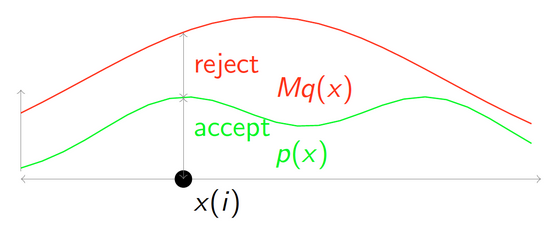然后重复如下步骤，直到获得$m$个被接受的采样点：

• $q(x)$中获得一个随机采样点$x(i)$
• 对于$x_i$计算接受概率（acceptance probability） $\displaystyle \alpha = \frac{p(x_i)}{Mq(x_i)}$
• 从 Uniform(0,1) 中随机生成一个值，用$u$表示
• 如果$\alpha \geq u$，则接受$x_i$作为一个来自$p(x)$的采样值，否则就拒绝$x_i$并回到第一步

当然可以采用严密的数学推导来证明Reject Sampling的可行性。但它的原理从直观上来解释也是相当容易理解的。你可以想象一下在上图的例子中， 从哪些位置抽出的点会比较容易被接受。显然，红色曲线和绿色曲线所示之函数更加接近的地方接受概率较高，也即是更容易被接受，所以在这样的地方采到的点就会比较多， 而在接受概率较低（即两个函数差距较大）的地方采到的点就会比较少，这也就保证了这个方法的有效性。

为了验证，我们还是以本文最开始给出的那个分段函数$f(x)$为例来演示 Reject Sampling 方法。如下面图所示，参考分布我们选择的是均匀分布 （你当然可以选择其他的分布，但采用均匀分布显然是此处最简单的处理方式）。而且令常数$M=3$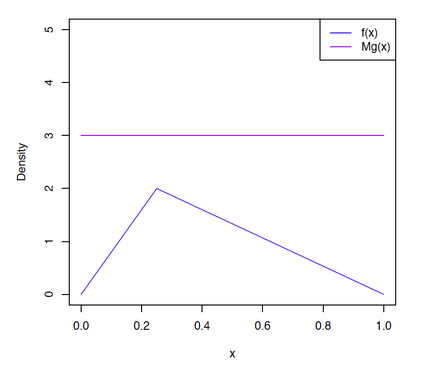得到的结果如下：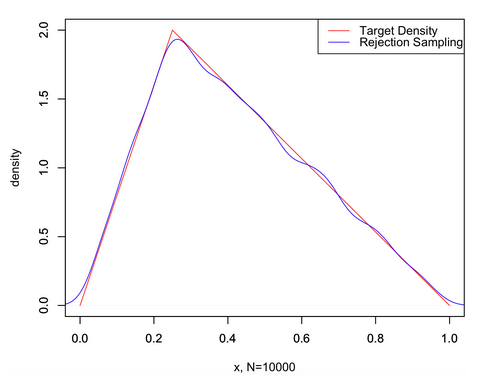从实验结果来看，采样还是很可观的。

# 总结

拒绝采样对于概率分布函数难以求解的数据进行采样是有效的，现在计算机的计算能力如此发达的情况下，更是有利于蒙特卡罗采样的发展。